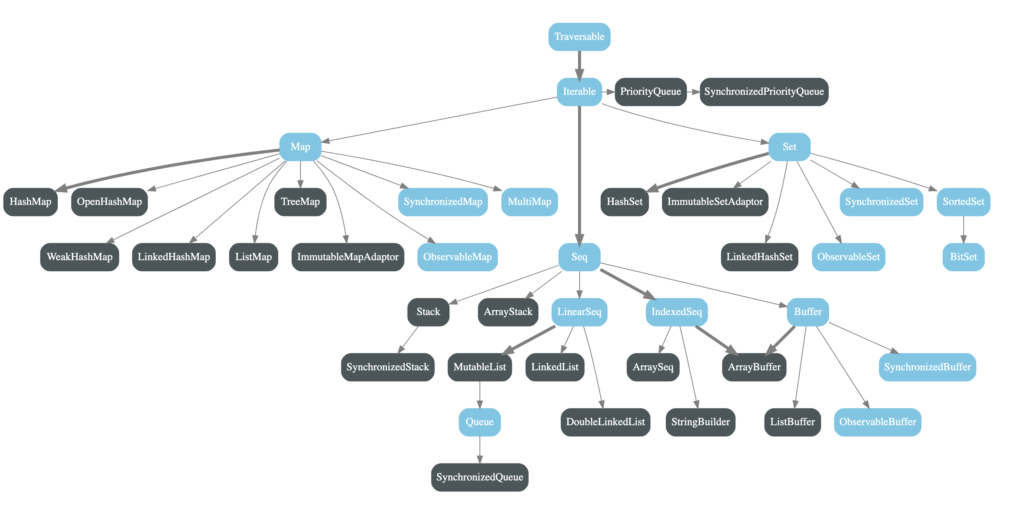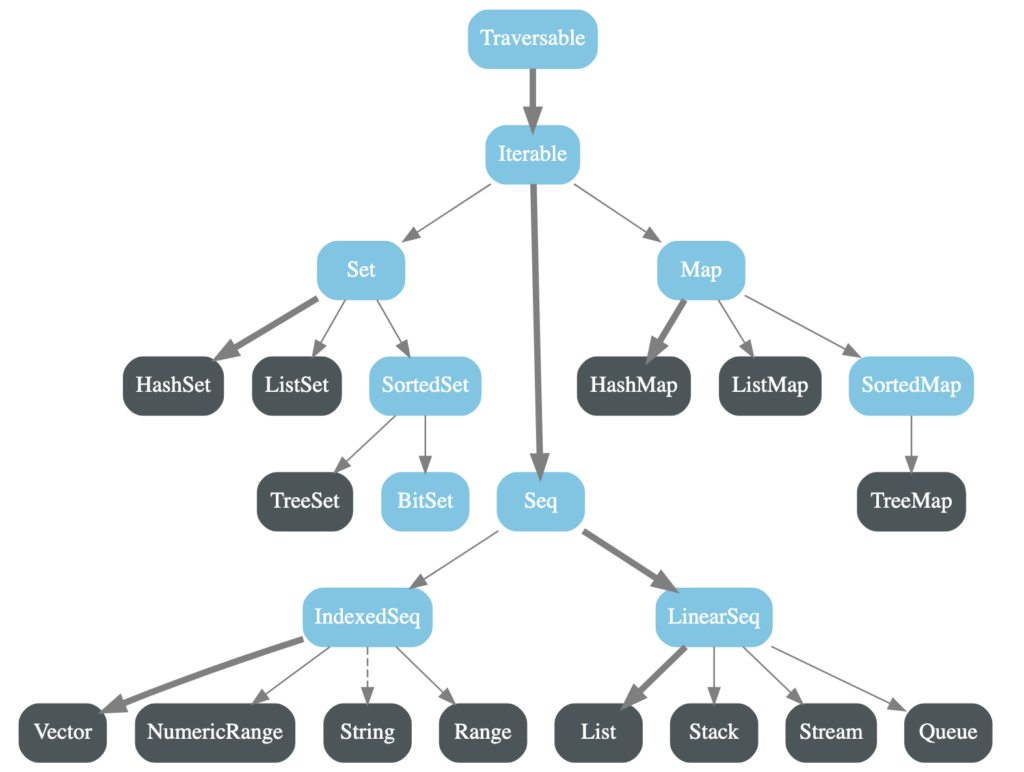Tutorials Hut

• # Scala Collections: List, Map, Set, Sequence and more

Scala collection is a collection of real world data structures like list, map, queue etc. with a rich set of methods. Scala collection is divided into two parts:

1. Mutable collection – This collection is mutable, can mutate or change the value. The package for mutable is scala.collection.mutable.

2. Immutable collection – This collection is immutable and values can’t be changed or mutated. The package for immutable is scala.collection.immutable.

Below diagram shows mutable Scala Collection:Below diagram shows immutable Scala Collection:## An Example Of Different Data Types in Scala Collection

```import java.awt.Color
import scala.collection.mutable
object Collections {

Traversable(1, 2, 3)

Iterable("x", "y", "z")

Map("x" -> 24, "y" -> 25, "z" -> 26)

Set(Color.red, Color.green, Color.blue)

mutable.SortedSet("hello", "world")

mutable.Buffer("x", "y", "z")

IndexedSeq(1.0, 2.0)

mutable.LinearSeq("a", "b", "c")

List(1, 2, 3)

mutable.HashMap("x" -> 24, "y" -> 25, "z" -> 26)
}```

Let’s Look into few import collection data types and classes and their methods.

## List In Scala

As the name suggests, the list holds a list of data inside it. It is one of the most popular collections in Scala and also in Java world. It is heavily used to store lists of different data types and perform different operations on them.

## Declaring The List

Below are different types of list created and with different styles.

Example:

```object ListInScala {

case class Car(number: String)
def main(args: Array[String]): Unit = {
//List of Int
val list: List[Int] = List(1, 2, 3, 4, 5)

//List of String
val listString: List[String] = List("Rom", "John", "Li")

//List of pojo objects

//Empty list

val myEmptyList: List[Nothing] = List.empty

//List of list
val myListOfList: List[List[Int]] = List(List(1, 2, 3), List(10,20,30))

//List of String using using :: operator

val myStringList: List[String] = "apples" :: "oranges" :: "pears" :: Nil
}
}```

## Useful Methods of Scala List With Example

Let’s look into head, isEmpty, tail, and other useful methods in the list.

• head – This method returns the first element of list
• tail – This method returns the last element of the list.
• isEmpty – Checks if the list is empty or not.
• nonEmpty – Checks if the list is non empty.
• take: Picks the elements of the list and creates another list.
• last: Picks the last element of the list.
• takeRight: Returns list by selecting elements from right.
• reverse: Reverses the list
• foreach: Iterates of each element of the list.

Example:

Below class shows how to use common methods of list.

```object ListInScala {

case class Car(number: String)

def main(args: Array[String]): Unit = {

val list: List[Int] = List(10, 20, 30, 40)

println("tail: "+list.tail)

println("isempty: "+ list.isEmpty)

println("take: "+list.take(1))

println("last: "+list.last)

println("nonEmpty: "+ list.nonEmpty)

println("takeRight: "+list.takeRight(3))

println("reverse: "+list.reverse)

println(list.foreach(data => println("element in list: "+data)))
}
}```

Output

tail: List(20, 30, 40)

isempty: false

take: List(10)

last: 40

nonEmpty: true

takeRight: List(20, 30, 40)

reverse: List(40, 30, 20, 10)

element in list: 10

element in list: 20

element in list: 30

element in list: 40

()

## Using filter and filterNot in List

We can use filter method to filter list by passing a lambda function which returns Boolean, similarly we can use filterNot to not filter based on passed condition.

Example:

`object ListMoreExamples {  def main(args: Array[String]): Unit = {  val list = List(10, 20, 30, 40)  println(list.filter(data => data>20))  println(list.filterNot(data => data > 20))  }}`

Output

`List(30, 40)List(10, 20)`

## Using map in The List

Using map function on List we can change the value of each element by performing some operation or even transform from one type to another.See below example where we manipulated values of list and also transformed from Int to String.

Example 1:

`object ListMoreExamples {  def main(args: Array[String]): Unit = {  val list = List(10, 20, 30, 40)    println("Double each element in list using map: "+list.map(data => data*2))    println("Convert each element in list to String with diff value using map: "+list.map(data => "Converted to String:"+data)) }}`

Output:

`Double each element in list using mapList(20, 40, 60, 80)Convert each element in list to String with diff value using mapList(Converted to String:10, Converted to String:20, Converted to String:30, Converted to String:40)`

Example 2:

In this example we will see how we can use map to do a lot of manipulation and then produce a completely new result. In this example we took a list of Student objects and converted with new results to GradeOfStudent objects.

```object ListMoreExamples {
case class Student(rollNum: Int,marks: Int, name: String)

def main(args: Array[String]): Unit = {
val studentList = List(Student(100, 99, "John"), Student(101, 66, "Rom"), Student(102, 60, "Li"), Student(100, 50, "Monty"))

//Use map and grade A for marks > 80 , B for marks > 60 < 80, C for marks > 60
val gradeOfStudentList = studentList.map(student => {

if(student.marks >= 80) {

} else if(student.marks < 80 && student.marks >= 60) {

}else {

}

})

println("Student List: "+studentList)

}
}```

Output

`Student List: List(Student(100,99,John), Student(101,66,Rom), Student(102,60,Li), Student(100,50,Monty))GradeOfStudent: List(GradeOfStudent(100,A), GradeOfStudent(101,B), GradeOfStudent(102,B), GradeOfStudent(100,C))`

## Sequence In Scala

Sequence in Scala is similar to a list where we have a sequence or list of some data and we can perform many operations on it by using methods of sequence. Most of the methods in list and sequence are similar.

Example of sequence and its methods:

```object SequenceInScala {
case class Car(number: String)
def main(args: Array[String]): Unit = {
//Seq of Int
val seqExample: Seq[Int] = Seq(1, 2, 3, 4, 5)
//Seq of String

val listString: Seq[String] = Seq("Rom", "John", "Li")

//Seq of pojo objects

//Empty Seq
val myEmptyList: Seq[Nothing] = Seq.empty

//Seq of Seq
val myListOfList: Seq[Seq[Int]] = Seq(Seq(1, 2, 3), Seq(10,20,30))

//Seq of String using using :: operator

val myStringList: Seq[String] = "apples" :: "oranges" :: "pears" :: Nil

val seq: Seq[Int] = Seq(10, 20, 30, 40)

println("tail: "+seq.tail)

println("isempty: "+ seq.isEmpty)

println("take: "+seq.take(1))

println("last: "+seq.last)

println("nonEmpty: "+ seq.nonEmpty)

println("takeRight: "+seq.takeRight(3))

println("reverse: "+seq.reverse)

println(seq.foreach(data => println("element in seq: "+data)))
}
}```

Output:

tail: List(20, 30, 40)

isempty: false

take: List(10)

last: 40

nonEmpty: true

takeRight: List(20, 30, 40)

reverse: List(40, 30, 20, 10)

element in seq: 10

element in seq: 20

element in seq: 30

element in seq: 40

()

## Set In Scala

Set is similar to Java collection set, it always holds a unique set of values, if you add any duplicate it will just replace the old one and not create a new element in set.

Example of Scala Set and Useful methods:

```object SetsExampleobject {

case class Car(number: String)

def main(args: Array[String]): Unit = {

//Set of Int
val list: Set[Int] = Set(1, 2, 3, 4, 5)

//Set of String
val setStringList: Set[String] = Set("Rom", "John", "Li")

//Set of pojo objects

//Empty set
val emptySet: Set[Nothing] = Set.empty

//Set of sets
val setOfSets: Set[Set[Int]] = Set(Set(1, 2, 3), Set(10,20,30))

val set: Set[Int] = Set(10, 20, 30, 40)

println("tail: "+set.tail)
println("isempty: "+ set.isEmpty)
println("take: "+set.take(1))
println("last: "+set.last)
println("nonEmpty: "+ set.nonEmpty)
println("takeRight: "+set.takeRight(3))
println(set.foreach(data => println("element in set: "+data)))
//Find min max in set
}
} ```

Output:

tail: Set(20, 30, 40)

isempty: false

take: Set(10)

last: 40

nonEmpty: true

takeRight: Set(20, 30, 40)

element in set: 10

element in set: 20

element in set: 30

element in set: 40

()

## Map In Scala

Scala Map is similar to Java map, it is based on key value pairs, each map element will have one key and against it one value.

• Each element in the map is a pair of keys and values against that key.
• Map can’t hold duplicate keys.
• If we want to update or change any value in a map we can do it by using a key.

Example of Scala Map and Useful Methods:

```object MapExampleInScala {

def main(args: Array[String]): Unit = {

var myMap: Map[String, String] = Map("101" -> "Rom", "102" -> "Joo", "103" -> "Sandy")

println("contains: "+myMap.contains("101"))

println("isEmpty: "+myMap.isEmpty)

println("nonEmpty: "+myMap.nonEmpty)

println("take: "+myMap.take(2))

println("takeRight: "+myMap.takeRight(2))

println("partition: "+myMap.partition(data => data._1 == "102"))

myMap += ("104" -> "new guy")

//find value for a given key

println(myMap("104"))

//Filter map

println(myMap.filter(data => data._1 == "101"))

//map on Map and get values in list

println(myMap.map(data => data._2+" is good"))
}
}```

Output

contains: true

isEmpty: false

nonEmpty: true

head: Map(102 -> Joo, 103 -> Sandy)

take: Map(101 -> Rom, 102 -> Joo)

takeRight: Map(102 -> Joo, 103 -> Sandy)

partition: (Map(102 -> Joo),Map(101 -> Rom, 103 -> Sandy))

new guy

Map(101 -> Rom)

List(Rom is good, Joo is good, Sandy is good, new guy is good)

## Next Article

• Scala Future